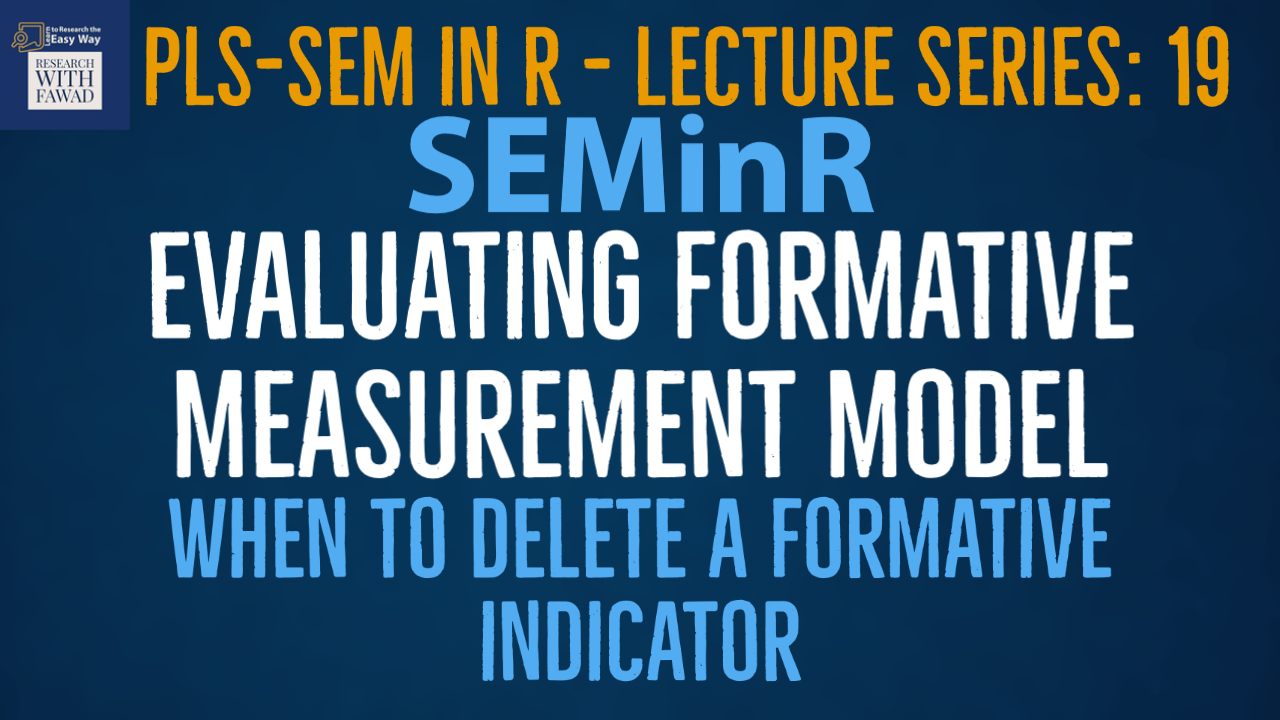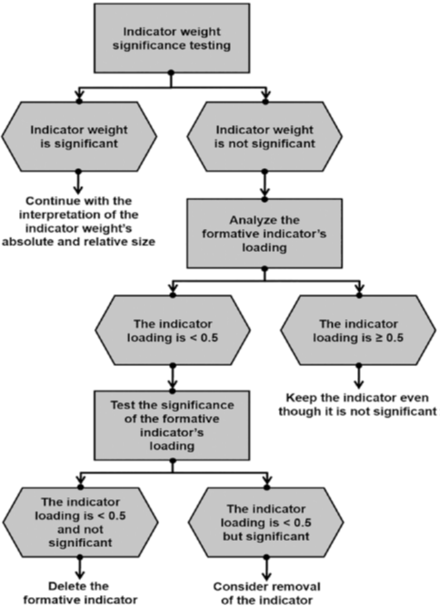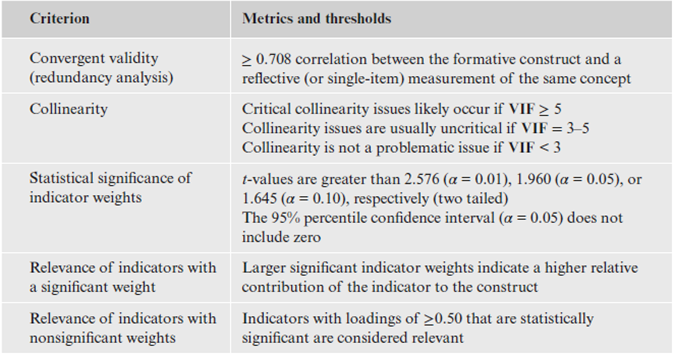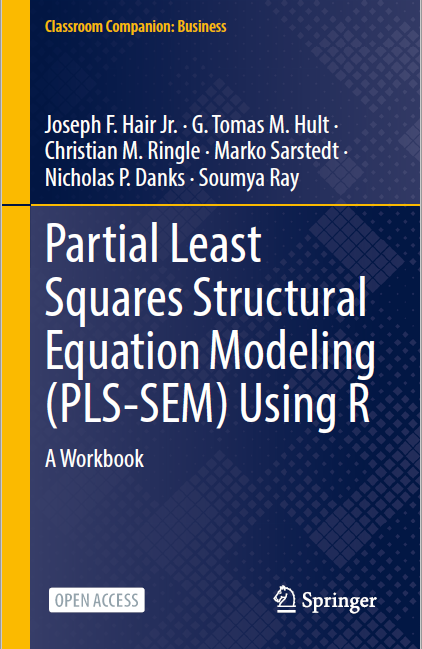# Evaluating Formative Measurement Model - Step 3: Indicator Weights### SEMinR Lecture Series

This session compliments the step 3 of the formative measurement model assessment. The tutorial will guide on deciding when to delete a formative indicator.

## Formative Model Assessment

• When deciding whether to delete formative indicators based on statistical outcomes, researchers need to be cautious for the following reasons.
• First, formative indicator weights are a function of the number of indicators used to measure a construct. The greater the number of indicators, the lower their average weight.
• Formative measurement models are inherently limited in the number of indicator weights that can be statistically significant.
• Second, indicators should seldom be removed from formative measurement models since formative measurement requires the indicators to fully capture the entire domain of a construct, as defined by the researcher in the conceptualization stage.
• In contrast to reflective measurement models, formative indicators are not interchangeable, and removing even one indicator can therefore reduce the measurement model’s content validity (Bollen & Diamantopoulos, 2017).
• Important: Formative indicators with nonsignificant weights should not automatically be removed from the measurement model, since this step may compromise the content validity of the construct.## Summary Formative Model Assessment

• After the statistical significance of the formative indicator weights has been assessed, the final step is to examine each indicator’s relevance. With regard to relevance, indicator weights are standardized to values between −1 and +1.
• Thus, indicator weights closer to +1 (or −1) indicate strong positive (or negative) relationships, and weights closer to 0 indicate relatively weak relationships.
• Table summarizes the rules of thumb for formative measurement model assessment.## Complete Code

``````library(seminr)
# Create measurement model
simple_mm <- constructs(
composite("Vision", multi_items("VIS", 1:4), weights = mode_B),
composite("Development", multi_items("DEV", 1:7), weights = mode_B),
composite("Rewards", multi_items("RW",1:4), weights = mode_B),
composite("Collaborative Culture", multi_items("CC", 1:6)))
# Create structural model
simple_sm <- relationships(paths(from = c("Vision", "Development", "Rewards"), to = "Collaborative Culture"))
# Estimate the model
simple_model <- estimate_pls(data = datas, measurement_model = simple_mm, structural_model = simple_sm, missing = mean_replacement, missing_value = "-99")
# Summarize the model results
summary_simple <- summary(simple_model)
#Descriptive Stattistics Summary
summary_simple\$descriptives\$statistics
# Iterations to converge
summary_simple\$iterations
# Collinearity analysis
summary_simple\$validity\$vif_items
# Bootstrap the model on the PLS Estimated Model
boot_model <- bootstrap_model(
seminr_model = simple_model,
nboot = 1000, cores = parallel::detectCores(), seed = 123)
# Store the summary of the bootstrapped model
# alpha sets the specified level for significance, i.e. 0.05
summary_boot <- summary(boot_model, alpha = 0.05)
# Inspect the bootstrapping results for indicator weights
summary_boot\$bootstrapped_weights

## Reference

Hair Jr, J. F., Hult, G. T. M., Ringle, C. M., Sarstedt, M., Danks, N. P., & Ray, S. (2021). Partial Least Squares Structural Equation Modeling (PLS-SEM) Using R: A Workbook.The tutorials on SEMinR are based on the mentioned book. The book is open source and available for download under this link.